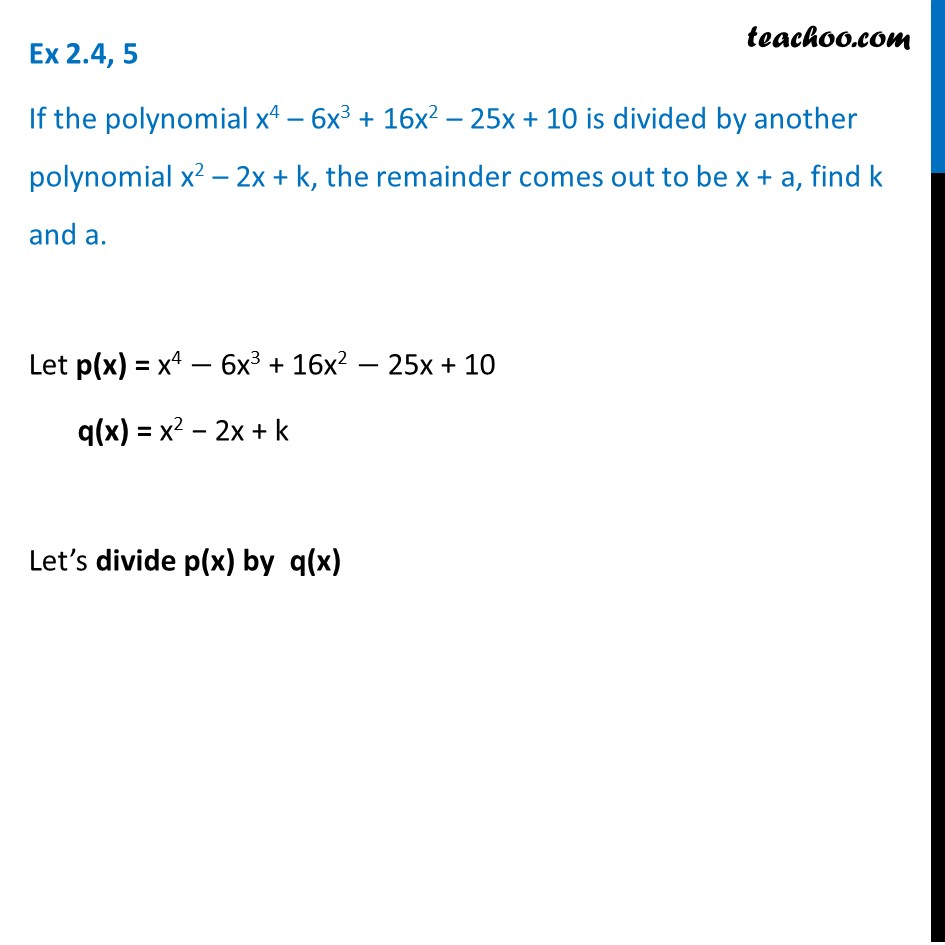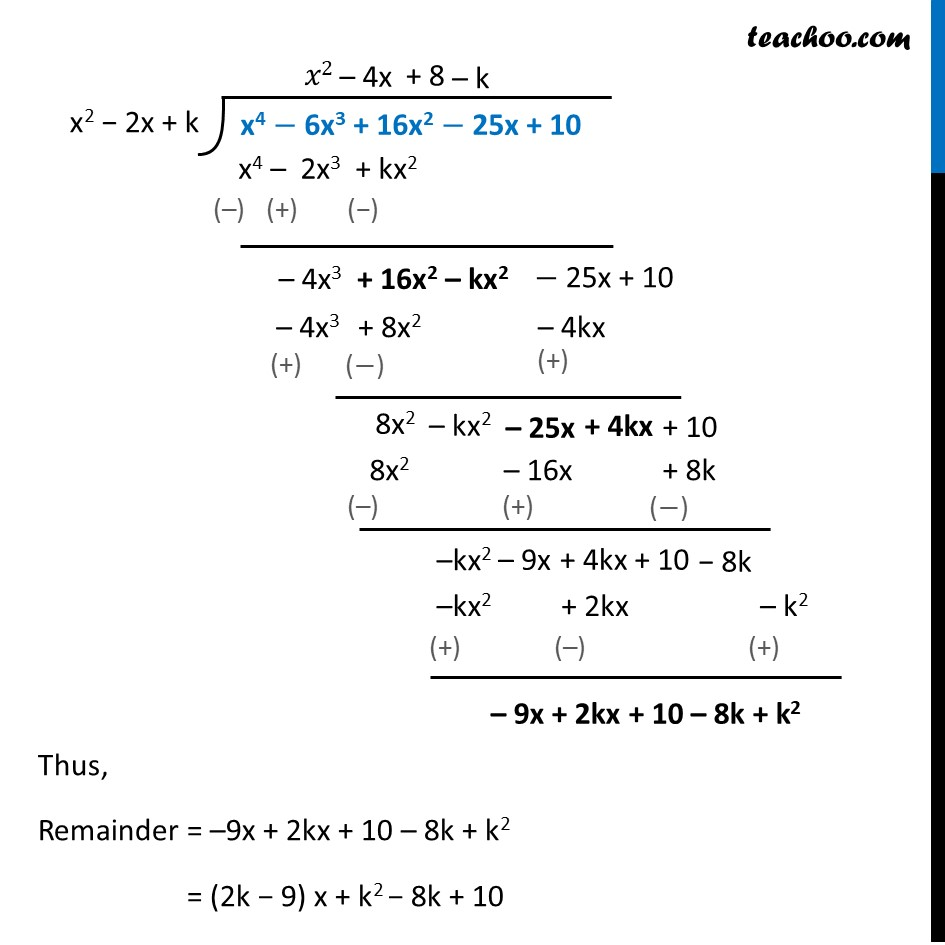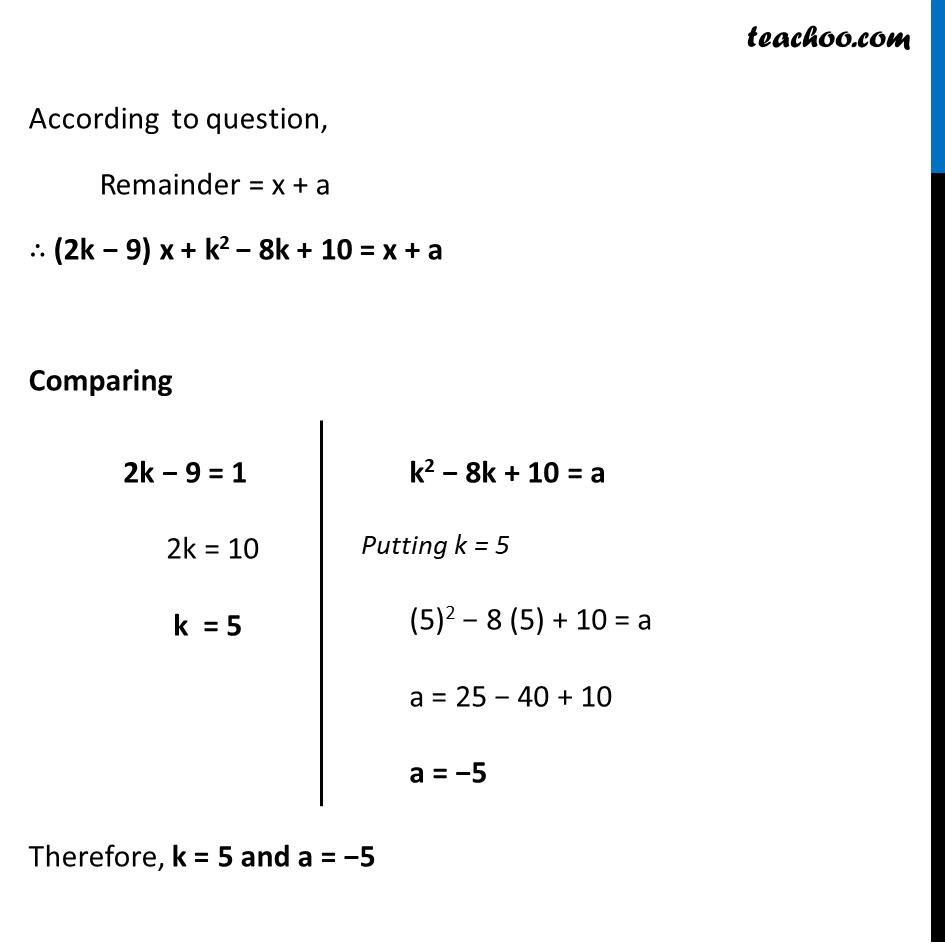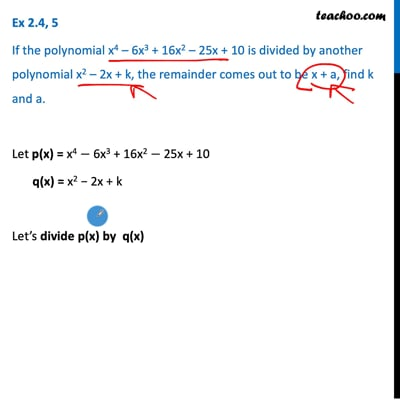Ex 2.4 (Optional)

Chapter 2 Class 10 Polynomials
Serial order wiseThis video is only available for Teachoo black users

Introducing your new favourite teacher - Teachoo Black, at only ₹83 per month

### Transcript

Ex 2.4, 5 If the polynomial x4 – 6x3 + 16x2 – 25x + 10 is divided by another polynomial x2 – 2x + k, the remainder comes out to be x + a, find k and a. Let p(x) = x4 − 6x3 + 16x2 − 25x + 10 q(x) = x2 − 2x + k Let’s divide p(x) by q(x) x2 − 2x + k x4 − 6x3 + 16x2 − 25x + 10 Thus, Remainder = –9x + 2kx + 10 – 8k + k2 = (2k − 9) x + k2 − 8k + 10 According to question, Remainder = x + a ∴ (2k − 9) x + k2 − 8k + 10 = x + a Comparing Therefore, k = 5 and a = −5 2k − 9 = 1 2k = 10 k = 5 k2 − 8k + 10 = a Putting k = 5 (5)2 − 8 (5) + 10 = a a = 25 − 40 + 10 a = −5